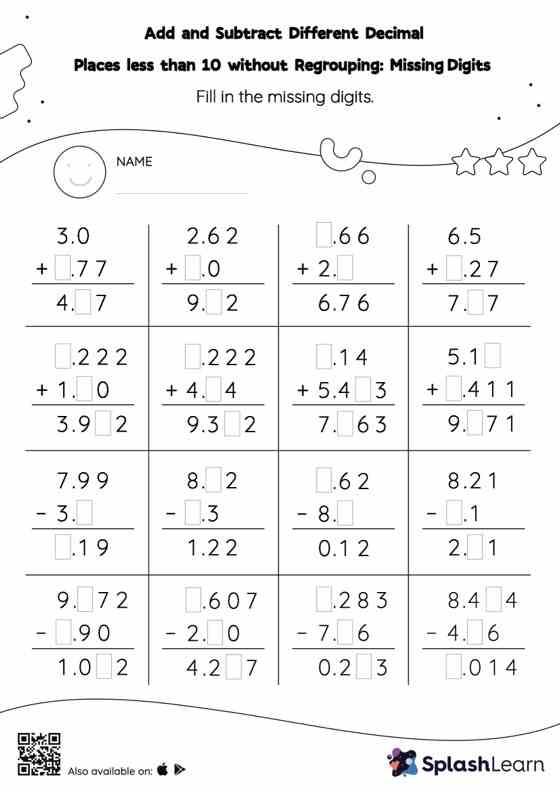# Add and Subtract Different Decimal Places less than 10 without Regrouping: Missing Digits Worksheet

Home > Add and Subtract Different Decimal Places less than 10 without Regrouping: Missing DigitsThis add and subtract different decimal places less than 10 without regrouping worksheet consists of tasks to help your young mathematician develop fluency with addition and subtraction. Students use the relationship between addition and subtraction to find the missing number while adding and subtracting decimals. They do not need to regroup the numbers in add and subtract different decimal places less than 10 without regrouping worksheet to get to the result.